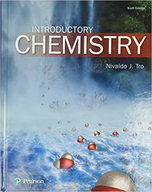×
Get Full Access to Introductory Chemistry (Masteringchemistry) - 6 Edition - Chapter 1 - Problem 5
Get Full Access to Introductory Chemistry (Masteringchemistry) - 6 Edition - Chapter 1 - Problem 5

×ISBN: 9780134302386 2037

## Solution for problem 5 Chapter 1

Introductory Chemistry (MasteringChemistry) | 6th Edition

• Textbook Solutions
• 2901 Step-by-step solutions solved by professors and subject experts
• Get 24/7 help from StudySoup virtual teaching assistantsIntroductory Chemistry (MasteringChemistry) | 6th Edition

4 5 1 261 Reviews
14
0
Problem 5

Define chemistry.

Step-by-Step Solution:
Step 1 of 3

Avogadro’s Number: One Mole  Chemicals often use a measuring unit called the mole  Mole or Avogadro’s number is defined as o 1 Mole of atoms= 6.022 x 10^23 atoms Counting Atoms Using Moles  A mole happens to be the same number of atoms present in a sample that weighs the element’s atomic weight  Ex. 6.022 x 10^23 atoms of zinc weighs 65.39 g  Ex. 6.022 x 10^23 atoms of magnesium weighs 24.305 g Molar Mass  Molar mass of an element is mass in grams of one mole of atom of the element  One mole of element has mass in grams equal to its atomic weight  1 mole of Fe has 6.022 x 10^23 number of atoms and weighs 55.847 g  1 mole of Al has 6.022 x 10^23 number of atoms and weighs 26.98 g  Ex. How many atoms are in 5.5 moles of atoms o 5.5 mole x (6.022 x 10^23 atoms/1 mole) = 2.3 x 10^24 atoms  Ex. How many moles of Cs are there in a sample that contains 6.21 x 10^22 Cesium atoms o 6.21 x 10^22 atoms x (1 mole/6.022 x 10^23 atoms) = 0.102 mole Cs  What is the mass in grams of 4.30 moles of Al o Mass of Al: 26.98 g/mol o 4.30 mole of H x (26.98 g/1 mole) = 196 g of Al  How many moles are present in 1.00 g of Fe o M = 55.8 g/mol o 1.00 g Fe x (1 mol/55.8 g) = 0.0179 mol of Fe  You are given 1.0 g samples of He, Fe, Li, Si and C. Which sample contains the largest number of atoms o He; a decrease in atomic weight → increase in number of atoms

Step 2 of 3

Step 3 of 3

##### ISBN: 9780134302386

Introductory Chemistry (MasteringChemistry) was written by Aimee Notetaker and is associated to the ISBN: 9780134302386. Since the solution to 5 from 1 chapter was answered, more than 205 students have viewed the full step-by-step answer. The full step-by-step solution to problem: 5 from chapter: 1 was answered by Aimee Notetaker, our top Chemistry solution expert on 04/04/22, 10:38AM. This full solution covers the following key subjects: . This expansive textbook survival guide covers 19 chapters, and 2612 solutions. The answer to “?Define chemistry.” is broken down into a number of easy to follow steps, and 2 words. This textbook survival guide was created for the textbook: Introductory Chemistry (MasteringChemistry), edition: 6.

## Discover and learn what students are asking

Calculus: Early Transcendental Functions : Second-Order Homogeneous Linear Equations
?Finding a General Solution In Exercises 5-30,find the general solution of the linear differential equation. y’’ + 2y' = 0

Calculus: Early Transcendental Functions : Second-Order Nonhomogeneous Linear Equations
?Finding a Particular Solution In Exercises 5-10,find a particular solution of the differential equation. y’’ - y’ - 6y = 4

Statistics: Informed Decisions Using Data : Bias in Sampling
?Why is it rare for frames to be completely accurate?

Statistics: Informed Decisions Using Data : Applications of the Normal Distribution
?Explain why P(X ? 220) should be reported as > 70.9999 if X is a normal random variable with mean 100 and standard deviation 15.

Statistics: Informed Decisions Using Data : Estimating a Population Standard Deviation
?True or False: The chi-square distribution is symmetric.

Unlock Textbook Solution

Enter your email below to unlock your verified solution to: